Trial ends in
JoVE Science Education
Mechanical Engineering

A subscription to JoVE is required to view this content.
You will only be able to see the first 20 seconds.

Conservation of Energy Approach to System Analysis
Science Education (Mechanical Engineering)
Mass Conservation and Flow Rate Measurements
Science Education (Mechanical Engineering)
Heat Exchanger Analysis

Conservation of Energy Approach to System Analysis

Overview

Source: Ricardo Mejia-Alvarez and Hussam Hikmat Jabbar, Department of Mechanical Engineering, Michigan State University, East Lansing, MI

The purpose of this experiment is to demonstrate the application of the energy conservation equation to determine the performance of a flow system. To this end, the energy equation for steady, incompressible flow is applied to a short pipe with a gate valve. The gate valve is then gradually closed and its influence on flow conditions is characterized. In addition, the interplay between this flow system and the fan that drives the flow is studied by comparing the system curve with the characteristic curve of the fan.

This experiment helps understanding how energy dissipation is used by valves to restrict the flow. Also, under the same principle, this experiment offers a simple method to measure flow rate using the pressure change across a sharp entrance.

Principles

Analysis of fluid flow is frequently carried out based on how energy transforms as the fluid flows through a system. The total energy carried by a flow includes kinetic, potential, and thermodynamic energy. These forms of energy may transform into each other freely, and the energy contained in the flow at a given position in a flow system is the balance between the total incoming energy, the added energy, and the subtracted energy. This energy balance can be expressed in equation form as follows [1, 2]: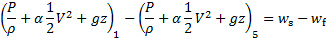(1)

Here, the subindices 1 and 5 refer to inlet and outlet conditions of the flow system respectively. Figure 2(A) shows a schematic of the flow system: the air enters through a flow conditioning system, or contraction, called plenum (its discharge shown in Figure 3(A)). Then, it transitions to a short pipe with a valve at its end (see Figure 2(B) for details of the pipe/valve system, and Figures 2(A) and (B) for pictures). The air discharges from the valve into an enclosed space isolated from the exterior called "receiver" (see Figure 3 for reference). As shown in Figure 3, the receiver is large enough to house the data acquisition system and the experimentalists. Finally, the flow exits the receiver through a perforated plate that serves as a damper for flow regulation (see Figure 3(C) for reference). The air is then captured by a centrifugal fan that discharges back to the atmosphere. The terms between parenthesis represent the specific energy content at each port, which is the sum of gravitational potential energy, kinetic energy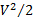, and thermodynamic (or pressure potential) energy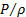. The coefficientis used to take into account the shape of the velocity profile. For the present experiment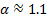because the flow is turbulent [1, 2]. As such, the left-hand side of equation (1) represents the change in energy content between the entry and exit ports. In the absence of external work or dissipative effects, this difference is zero. However, most engineering applications involving real flows include addition or subtraction of energy by means of flow machinery,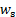, and dissipative effects,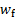. These two effects are included on the right-hand side of equation (1).

In this experiment, a centrifugal fan will be used to induce the flow, that is, to add energy to the fluid. For this kind of machine, the termis a function of the flow rate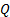called characteristic curve and is determined experimentally: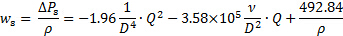(2)

Here,and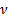are the density and kinematic viscosity of air at local conditions, and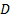is the diameter of the flow system (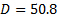mm in the present experiment). Equation (2) is in a form that ensures that the constants in the first and second term are dimensionless, while the constant in the third term is in units of pressure (Pa) to ensure dimensional homogeneity. Equation (2) is the “Fan performance curve” shown in Figure 1.

Finally, the energy dissipation is proportional to the kinetic energy of the flow: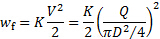(3)

Note that, by using the continuity equation (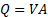, whereis the cross-sectional area [1, 2]), the energy dissipation can also be written in terms of the flow rate,. Figure 1 presents this last form of equation (3) as the "Flow system curve". In equation (3), the proportionality coefficient, is called the loss coefficient and is the addition of all dissipative effects induced by the elements of the flow system as a result of viscous interactions. While the contributions of pipes and ducts depend on their roughness, length, and Reynolds number, the contribution of pipe fittings, entrances and discharges, expansions, contractions, bends, and valves depends on their particular geometries. For the present experiment, the overall loss coefficient is the combination of an entrance, a valve, and a discharge: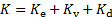(4)

Where the values of the loss coefficients for the entrance and discharge are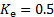and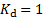respectively (see [1, 2] for reference). The loss coefficient for the valve,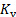, will be discussed in the following section.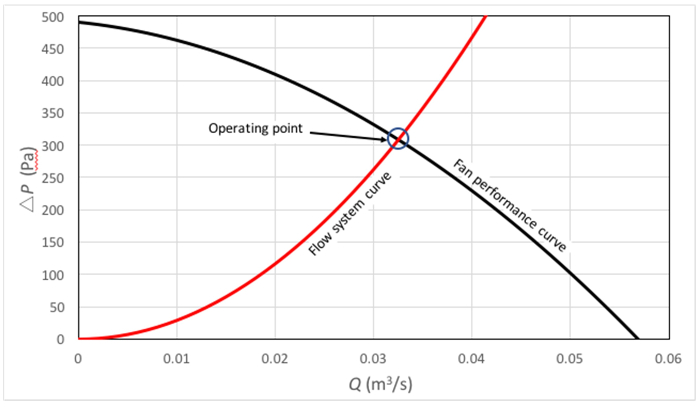Figure 1. Example of system curve and fan performance curve.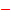: system curve;: fan performance curve. The blue circle highlights the intersection between both curves, which is the operating point.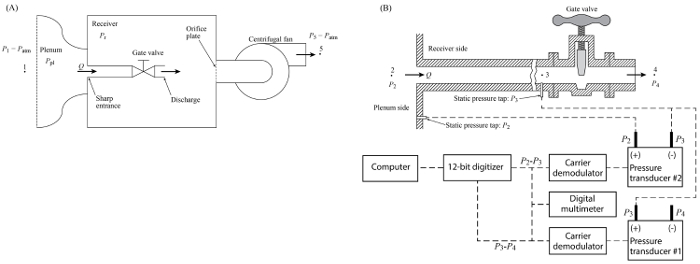Figure 2. Experimental setting. (A): Flow facility. The flow moves from left to right; it enters the plenum through a flow conditioning section, then flows through the pipe and valve, discharges inside the receiver, and finally exits the system through the fan. (B): Details of the flow system and data acquisition equipment. Please click here to view a larger version of this figure.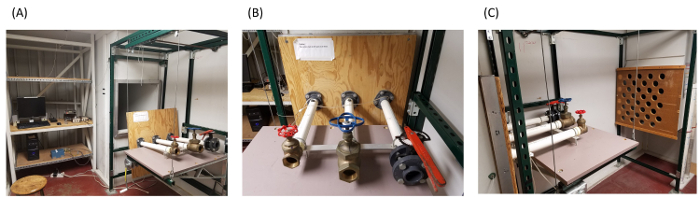Figure 3. Data acquisition system. (A) The connections of these data acquisition devices follow the diagram in Figure 2(B). (B) Virtual interface for data acquisition (written in LabView). Please click here to view a larger version of this figure.

Dissipation characteristics of a valve

Since valves are essentially devices of variable geometry, their specific loss coefficient is a function of the rotation angle of their shaft (). With this in mind,varies as the valve is opened or closed according to the following relationship: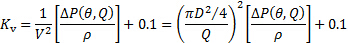(5)

Here, we used the continuity equation again to express the loss coefficient in terms of the flow rate. Furthermore, equation (5) suggests that we can determine the loss coefficient for the valve by knowing the value of the flow rate and the pressure drop across the valve as a function of the opening angle and flow rate: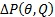. This is also the pressure difference between points 3 and 4 in Figure 2(B) (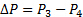).

Operating point

As shown in Figure 1, the Operating Point of a flow system driven by a flow machine, like the one featured in this experiment, is given by the intersection point of the characteristic curve of the fan and the flow-system curve. This point can be characterized using the energy equation as follows: as can be seen in Figure 2(A), air enters the flow system from a big room at atmospheric conditions and exits to the same room without any significant change in height. In consequence, pressure, velocity, and height conditions at the system's inlet and outlet (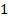and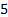subindices in equation (1) respectively) are identical and cancel each other. Consequently, the energy added by the fan is balanced by the energy dissipated by the flow system. In other words, equations (2) and (3) form an equality that results in the following relationship after simplification: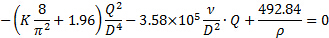(6)

The positive root of this equation expresses the Operating Point:(7)

Here, the sub-index "OP" stands for "Operating Point". The loss coefficient in equation (7) is the addition of the loss coefficients of the entrance, valve, and discharge. From equations (4) and (5):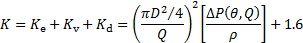(8)

Measurement of flow rate

Analogous to all the previous analyses, equation (1) is applied between the plenum and a point downstream of the sharp entrance (points 2 and 3 in figure 2(B)) to recover the following equation: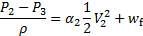(9)

Here, we used the fact that there is no change in height between points 2 and 3, and the velocity inside the plenum is negligible. Introducing the continuity equation (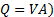and equation (3), we arrive at the following relationship for the flow rate in terms of the pressure difference between the plenum (point 2 in Figure 2(B)) and the static pressure upstream of the valve (point 3 in Figure 2(B)):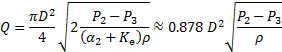(10)

The constant 0.878 is obtained after substituting for the values of the loss coefficient and velocity correction (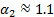and, see [1, 2] for reference) and grouping all the constants in a single one.

Procedure

1. Setting the facility

1. Make sure that the fan is not running, so there is no flow in the facility.
2. Verify that the data acquisition system (Figure 4(A)) follows the schematic in figure 2B.
1. Connect the positive port of pressure transducer #1 (see figure 2B for reference) to the pressure tap upstream of the valve (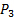).
2. Leave the negative port of the pressure transducer #1 open to the room conditions (receiver: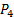). Hence, the reading of this transducer will be directly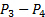.
3. Connect the positive port of pressure transducer #2 (see figure 2B for reference) to the plenum pressure tap ().
4. Connect the negative port of pressure transducer #2 (see figure 2B for reference) to the pressure tap upstream of the valve (). Hence, the reading of this transducer will be directly, as required by equation (10).
3. Make sure that the virtual channel 0 in the data acquisition system (Figure 4(B)) corresponds to pressure transducer #1 () and the virtual channel 1 corresponds to pressure transducer #2 ().
4. Set the data acquisition system to sample at a rate of 100 Hz for a total of 500 samples (i.e. 5s of data).

Table 1. Basic parameters for experimental study.

 Parameter Value Pipe diameter (D) 50.8 mm (2 inch) Transducer #1 calibration constant (m_p1) Pa/V Transducer #2 calibration constant (m_p2) Pa/V Local atmospheric pressure 100,474.15 Pa Local temperature 297.15 K Local air density 1.186 kg/m3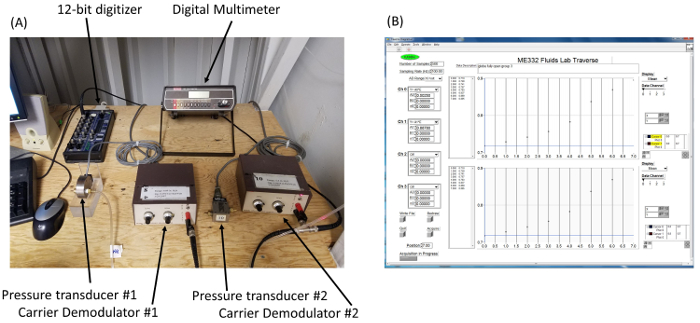Figure 4. Flow facility. (A): view of plenum discharge into the receiver section before installing the set of valves to be studied. (B): three different kind of valves inside the receiver. From left to right: gate valve, globe valve, butterfly valve. (C): exit ports from the receiver. The valves discharge the flow inside the receiver, and the fan sucks the flow out of the receiver through the perforated plate in the picture. Please click here to view a larger version of this figure.

2. Measurements

1. Record the diameter of the pipe connected to the valve and calculate its cross-sectional area.
2. Determine the maximum number of full turns of the handle required to move the valve from the fully closed position to the fully open position. If this number is not an integer, exclude the last fractional rotation to simplify this analysis. For the current experiment, the maximum number of full turns is 12.
3. Close the valve completely.
4. Rotate the handle of the valve until it is fully open while counting the number of full turns. For simplicity, use only an integer number of turns for the experiment. For example, it takes approximately 12 turns and 1/3 of a turn to fully open the valve used in this experiment. Hence, we will turn the handle of this valve only 12 full turns from its fully closed position and define that as the initial position (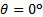).
5. Turn the flow facility on.
6. Use the data acquisition system to record the readings ofand.
7. Enter in table 2 the average values ofandobtained with the data acquisition system.
8. Close the valve by 1.5 turns.
9. Repeat steps 2.6 to 2.8 until table 2 is fully populated.
10. Turn the flow facility off.

3. Data Analysis

1. Determine the loss coefficient of the valve for each angular position using equation (5). Enter these values in table 2.
2. Determine the flow rate for each angular position of the valve using equation (10). Enter these values in table 2.
3. Determine the operating point using equation (7). Enter these values in table 2.
4. Calculate the relative difference between the measured flow rate and the operating point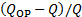5. Use equation (3) to produce a plot of the system curves for all the values of. Consider the total loss coefficient as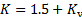.
6. Add the characteristic curve of the fan to this same plot using equation (2).

Table 2. Representative results. Measurements of pressure differences and estimations of flow rate and loss coefficients.

 [P_pl-P_a ] (Pa) [P_a-P_r ] (Pa) Q (m3/s) K Q_OP (m3/s) ε (%) 246.75 54.00 0.0327 0.450 0.0316 -3.16 208.62 114.22 0.0301 0.976 0.0293 -2.51 156.19 204.80 0.0260 2.198 0.0254 -2.30 109.30 281.69 0.0218 4.224 0.0214 -1.53 71.82 348.38 0.0176 7.863 0.0174 -1.26 38.72 408.60 0.0129 16.989 0.0128 -0.90 15.00 452.39 0.0081 48.359 0.0080 -0.32 2.51 482.50 0.0033 307.799 0.0033 -0.18

Conservation of energy is a well established physical principle that is frequently applied in the design and analysis of mechanical systems. Since energy is conserved, careful accounting of how it is added to and dissipated from a system as well as the internal transformations to the various forms can yield important details about the operating conditions. The advantage of this approach is that it often allows many details of the system to be ignored. So, the analysis can be greatly simplified. This video will illustrate the application of conservation of energy to a flow system with a gate valve. And show how this approach can be used to determine the operating point of the system as well as the loss coefficient of the valve.

Consider the flow facility shown in this schematic. Air is drawn into the plenum from atmospheric conditions and flows into the receiver room through a short pipe section with a sharp entrance, a gate valve, and an open discharge. The air then flows through an orifice plate and a centrifugal fan before returning to atmospheric conditions. The total energy carried by the flow is a combination of kinetic, potential, and thermodynamic components as shown in the equation for the specific energy at a point in the flow. These components can freely transform from one type to another through the system. Note that alpha is a correction factor to take into account that the velocity is not constant across the flow section. For turbulent flow, alpha is usually taken as one. And for laminar flows, it is noticeably larger. In pipe flows at moderate Reynolds numbers, alpha is approximately 1.1. Since energy is conserved, any difference in the specific energy between two points in the flow must be the result of external work on the fluid or dissipation. Additionally, if analysis is restricted to points at the same height, the gravitational potential will not contribute to the difference. This is the energy equation for the system. Now consider the system losses. The most significant losses will occur at the pipe entrance, the valve, and the discharge. These losses are proportional to the kinetic energy of the flow and can be related to the flow rate using continuity. It can be shown that the loss coefficient for the entrance and discharge are one half and one respectively. Consider what happens as the air flows from the plenum into the pipe section. No energy is added, but there is some dissipation at the entrance. Additionally, since the flow velocity in the plenum is negligible compared to the velocity in the pipe section, it can be ignored. The remaining terms can be rearranged to yield the flow rate in terms of the pressure difference between those points. Now consider the pressure drop from the pipe section upstream of the valve to the receiver. Again, no energy is added and losses will occur at the valve and discharge. Flow velocity in the receiver is negligible compared to the pipe section, so the equation simplifies again. In this case, the valve loss is a function of flow rate and the pressure difference can be determined. Finally, consider the whole system. The fluid enters and exits the system at the same pressure and velocity. So the work added by the shaft must be equal to the total losses in the system. If the performance curve of the fan is known, then the operating point, or expected flow rate of the system can be predicted for a given total loss factor. The operating point can be determined graphically by plotting the fan performance curve with the system performance curve. At a given flow rate, the fan curve represents the specific energy added in terms of a pressure jump, while the system curve represents the specific energy loss. At a steady state, these two contributions must be equal. Now that you understand how to use conservation of energy to analyze the system, let's use this technique to calibrate the valve and determine the operating point.

Before you begin setting up, familiarize yourself with the layout and safety procedures of the facility. Check that the fan is not running and there is no flow through the test area. Now set up the data acquisition system as shown in the diagram in the text. Connect the plenum pressure tab to the positive port of pressure transducer two. And then connect the pressure tab upstream of the valve to the negative port of transducer two as well as the positive port of transducer one. Leave the negative port of transducer one open to room conditions. The data acquisition software ensure that virtual channel zero and one correspond to pressure transducers one and two respectively. Finally, set the sampling rate to 100 hertz and total samples to 500. After the data acquisition system is set up, measure the inner diameter of the test pipe and calculate its cross sectional area. Next, turn the valve handle clockwise until the valve is completely closed. And then open the valve by one full turn of the handle at a time keeping count of the number of whole turns required to fully open the valve. If there is a partial turn remaining, return the handle to the nearest full turn. Pick a convenient increment based on the number of turns just counted. For example, if the number of turns was 12, an increment of 1.5 turns gives eight test points from fully open to almost fully closed. Leave the valve in the fully opened position and turn the flow facility on. Now, use the data acquisition system to determine the average pressure differences measured by both transducers at this valve position and record these values. Close the valve by one increment and repeat the measurement. Continue closing the valve by increments and taking measurements until the valve is almost fully closed. When all of the data has been collected, turn the flow facility off.

At each valve position measured by the number of turns from the fully opened position, you have a measurement of the pressure differences between the plenum and the pipe section upstream of the valve and the measurement of the pressure difference between the pipe section upstream of the valve and the receiver. Perform the following calculations for each position of the valve. First calculate the flow rate from the pressure drop between the plenum and the upstream pipe section using the equation derived earlier. Once the flow rate is known, the loss coefficient of the valve can be calculated from the pressure drop between the upstream pipe section and the receiver. Use the loss coefficient to determine the operating point or the expected air flow at this valve position. Finally, compare the operating point to the experimental flow rate by calculating the relative difference between the two. Now look at your results.

Plot the characteristic curve described in the text for the fan and then add the system curves for the total losses at each position of the valve. Both the slope of the system curve and the loss coefficient of the valve increases the valve is closed demonstrating an increase in energy dissipation as the flow is restricted. Conceptually, as KV approaches infinity, all of the energy is dissipated in the valve. In the range of flow rates observed, the percent error is low but always underestimated. Additionally, the error decreases as the valve is closed. This behavior is expected since the correction factor alpha increases slightly with the Reynolds number.

Conservation of energy is frequently used to analyze complex engineering systems. The kinetic energy carried by the wind can be harvest by wind turbines to produce electric power. By comparing upstream with downstream flow conditions, the energy equation can be used to assess how much energy has been removed from the wind. The magnitude of the energy recovered will be given by the shocked work. Change is gravitational potential energy can be used to assess the flow rate of water over a spillway. This is done in combination with the mass conservation equation by measuring the depths upstream and downstream of the spillway.

You've just watched the Jove's introduction to conservation of energy analysis. You should now understand how to apply an energy equation to a flow system, calibrate loss coefficients, and determine the operating point. Thanks for watching.

Results

Figure 5 shows the results for the current measurements. Here, the black solid line was generated with equation (2), and each red line with equation (3) for different values of the valve's loss coefficient. From the figure, it is evident that the system curve increases its slope as the valve closes. In other words, this experiment demonstrates that the principle behind the operation of a valve is to increase energy dissipation to restrict the flow. On the other hand, from equation (5), it could be inferred that the value ofbecomes infinity when the valve is fully closed. Conceptually, this condition means that all the energy is dissipated, hence completely impeding flow through the valve.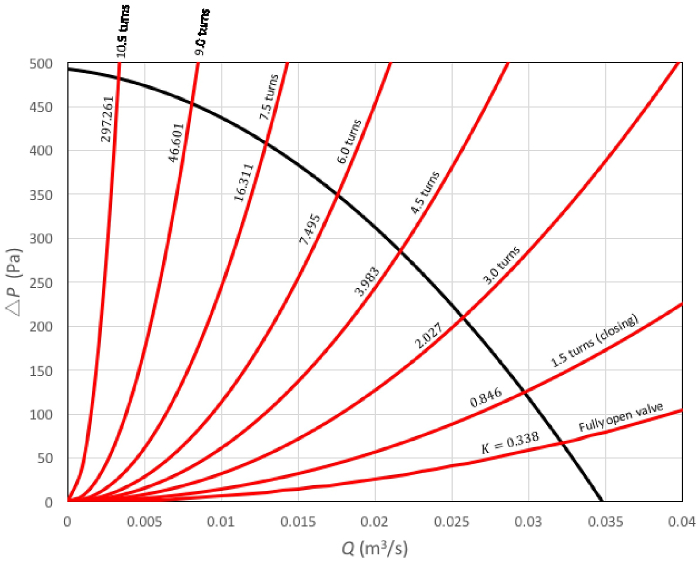Figure 5. Representative results.: system curves. Each curve in this family is the result of a different degree of valve opening. The slope of the curves increases as the valve is closed. Each curve has its correspondent loss coefficient for reference;: fan performance curve. Please click here to view a larger version of this figure.

As shown in Table 2, the error between the estimated flow rate at the operating point (equation (7)) and the measured flow rate (equation (10)) stays below 3.2% for the studied range of flow rates. Though this is a satisfactory result given the small percentage error, the estimated flow rate at the operating point is always underestimated, and follows a decreasing tendency as the valve is gradually closed. This tendency offers some insight into the behavior of the flow system, particularly because the value of the correction factor for velocity non-uniformityincreases slightly with Reynolds number. It is hence not surprising that the error increases with flow rate.

Applications and Summary

This experiment explored the application of the energy equation to characterize the action of a valve on pipe flow. It was observed that the valve induces flow resistance by increasing energy dissipation. Considering that the pressure drop along the flow system is directly proportional to the square of the flow rate, the effect of energy dissipation is captured by the magnitude of the proportionality coefficient. This coefficient is the addition of the loss coefficients of all the elements in the flow system, including the valve. The loss coefficient of the valve increases monotonically as the valve is gradually closed. And this effect increases the slope of the system curve as a result, reaching a value of infinity when the valve is fully closed.

The above described behavior was contrasted with the performance curve of the centrifugal fan that drives the flow. By comparing the flow rate measured directly with the flow rate estimated by the intersection of the system curve and the performance curve of the fan, it was demonstrated that this intersection point defines the operating conditions of flow rate and pressure demand.

This experiment served the purpose of demonstrating the principles of energy conservation to characterize a few different engineering applications: valve operation, flow rate measurement, and operating conditions of a flow system. Energy conservation can be used basically to characterize any flow system, these are two more examples of applications of the energy equation:

The kinetic energy carried by the wind can be harvested by wind turbines to produce electric power. By comparing upstream with downstream flow conditions, the energy equation can be used to assess how much energy has been removed from the wind. The magnitude of the energy recovered will be given by the shaft work,, in equation (1).

Change in gravitational potential energy can be used to assess the flow rate of water over a spillway. This is done in combination with the mass conservation equation by measuring the depths upstream and downstream of the spillway. For turbulent flow, the following equation would be a good approximation for the flow rate over the spillway: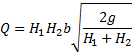(11)

Here,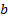is the channel's width and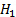and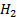are the upstream and downstream depths respectively.X